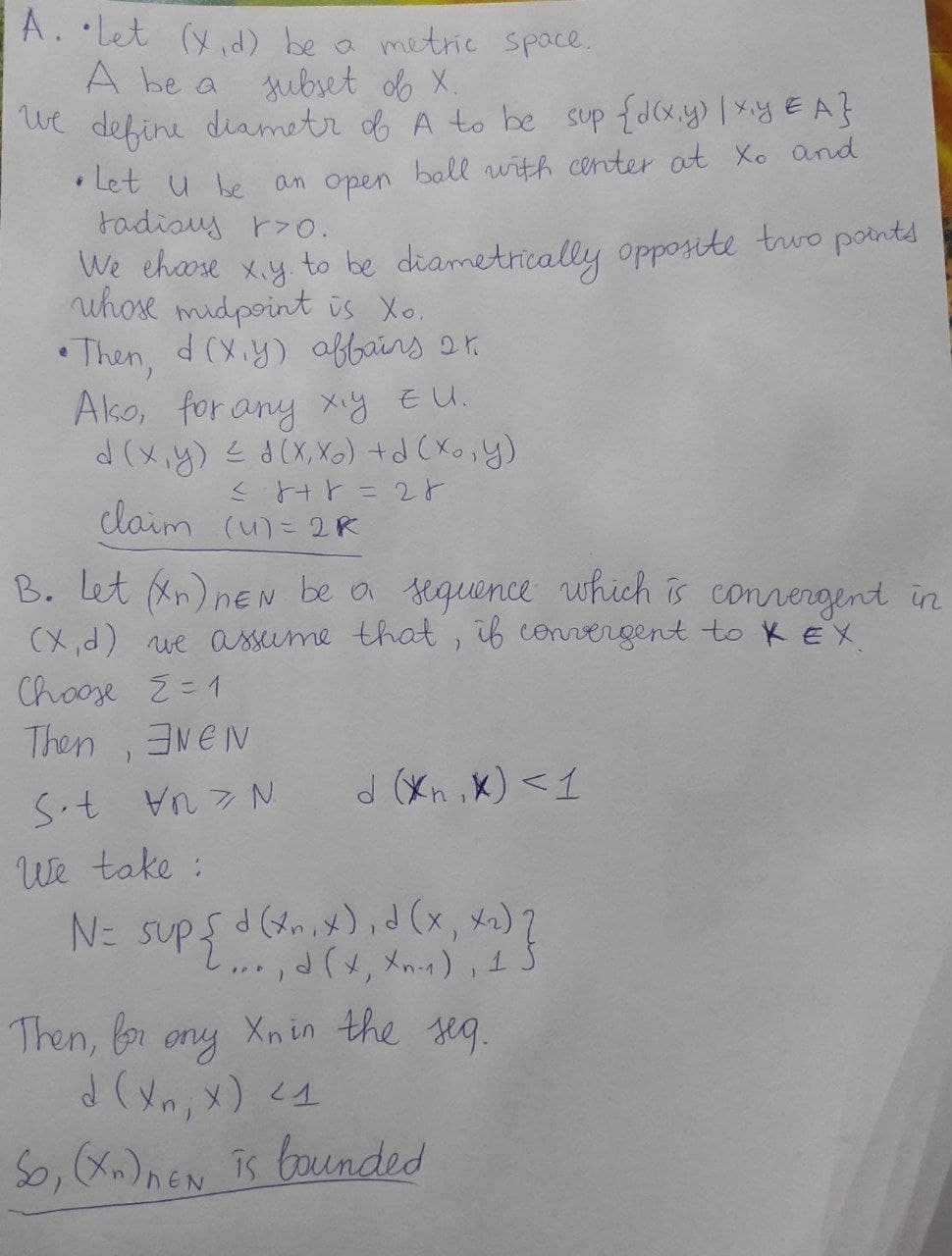Question# A. Let (X,d) be a metric space. Define a diametr of a ubset A of X and then the diametr of an open ball with center x_0 and rarios r>0. B. Use (A) to show that every covergence sequnce in (X,d) is bounded.

Analytic geometry
ANSWEREDA. Let (X,d) be a metric space. Define a diametr of a ubset A of X and then the diametr of an open ball with center $$\displaystyle{x}_{{0}}$$ and rarios r>0.